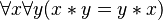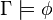Difference between revisions of "User:IssaRice/Computability and logic/Intended interpretation versus all interpretations"

Something I have found tricky in mathematical logic is that some theorems/propositions apply to just the intended/standard interpretation (structure), while others are about all possible interpretations. Texts also don't necessarily emphasize this point each time, so you have to figure it out.

Moral. Whenever you see the word "true" or "truth" in mathematical logic, always be sure whether it is about "truth in the intended interpretation" or "truth in all possible interpretations".

Normally when we say a mathematical statement is true, we mean that it is true in the standard (or intended) interpretation. For instance, we say that$2+2=4$ is true. But$2+2=4$ could also be false if we adopt a non-standard interpretation. For example, we could say "$2$" is the number zero, "$4$" is the number one, and "$+$" is the usual multiplication. In other words, we still keep the same signature ($2$ and$4$ are still constants,$+$ is still a binary function) so that the sentence is still grammatical, but we assign different meanings to these non-logical symbols. (Note: the equality symbol is usually taken to be special and cannot be assigned an arbitrary meaning.) A better example is a sentence like$\forall x \forall y (x*y = y*x)$, which can be true or false depending on whether a structure with a given operation is commutative.

When we talk about semantic consequence (a.k.a. logical consequence, logical implication) and write things like$\Gamma \models \phi$ (where$\Gamma$ is a set of sentences and$\phi$ is a sentence) then this doesn't just mean that "if all sentences in$\Gamma$ are true, then the sentence$\phi$ also is true". Rather, it means that this "if-then" is true in every possible interpretation. In other words, when we write$\Gamma \models \phi$ we mean that there is no interpretation in which every sentence in$\Gamma$ is true but$\phi$ is false. This is also sometimes expressed by saying that$\phi$ is true in all models of$\Gamma$.

Similarly when we say that a sentence is valid, this is much stronger than saying it is true in our intended interpretation. To say a sentence is valid means that no matter what interpretation we assign, the sentence ends up true. Thus,$2+2=4$ is not a valid sentence. But$2+2=4\text{ or }2+2\ne 4$ is a valid sentence; no matter what meanings we assigned to the non-logical symbols, the sentence would come out true. In other words, validity is the absence of a counterexample.

The soundness and completeness theorems are all about this "all possible interpretations" point of view. If a deductive system is sound and complete, then "true in all possible interpretations" exactly coincides with "is provable in the system".

Syntax doesn't seem to have this analogue. If something is provable, it automatically gets "true in all possible interpretations" (assuming soundness). There doesn't seem to be a way to partially prove something?

Soundness

• The soundness of a deductive system takes the "all possible interpretations" point of view.
• The soundness of a theory takes the "true in the intended interpretation" point of view.

Completeness

• The completeness of a deductive system (a.k.a. semantic completeness) takes the "all possible interpretations" point of view. Gödel's completeness theorem is about this form of completeness, so Gödel's completeness theorem is talking about all possible interpretations.
• The completeness of a theory (a.k.a. negation-completeness) is entirely syntactic, so it does not take either of the views (whenever we talk about truth, that is bringing in semantics). Gödel's first incompleteness theorem is about this form of completeness, so Gödel's first incompleteness theorem is talking about truth in the intended interpretation. -- actually this might be wrong. there is a way to phrase godel's incompleteness theorem in terms of models like "there is a sentence that is true in some models but not others"

Expresses, arithmetically defines

these are also relative to an interpretation.

Captures, defines

these are also relative to an interpretation.